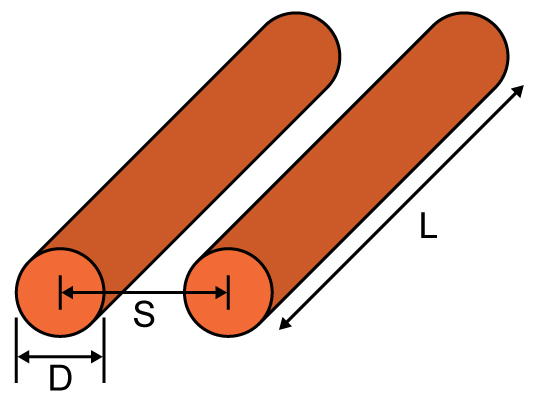# Parallel Wire Inductance Calculator

## This tool is designed to calculate the inductance of two parallel wires

H

### Overview

Inductance is the measure of how the magnetic flux and the current that created it, are linked. Any material that has current flowing through it and produces a magnetic flux has inductance. This tool calculates the inductance of a common object in electrical engineering: two parallel wires.

To use this tool, just fill in the required fields and click on the "calculate" button. Note: Copper, which most wires are made of, have a relative permeability approximately equal to 1.### Equation

$$L_{wires}\approx \frac{\mu _{o}\mu _{r}L}{\pi}\cosh^{-1}\left ( \frac{S}{D} \right )$$

Where:

$$S$$ = spacing between conductors (m)

$$D$$ = conductor diameter (m)

$$L$$ = length of conductor (m)

### Applications

Inductance is an important parameter that needs to be determined, especially in radio communications technology. Impedance (the measure of opposition to alternating current) of materials is a function of frequency, and for inductive materials, an increase in frequency means an increase of impedance. Knowing the inductance of materials means knowing their effects on the applied signal. When paired with stray capacitance at the right conditions, inductance can also result in resonance. This could result in an unwanted filter that could impede the signals propagating through a device.

1 Comment•tonigau April 20, 2020

This calc would be very handy, but may need some further work…
1.  trying to calc 16mm2 sq copper cable(2 core)
Distance = 5000mm, distance apart=3mm, entering diameter greater than 3mm gives NaN, dia=3 gives 0.00
2. The polarity of the current in each wire is not specified. Is it a 2 core cable (+ & -) source to load, or 2 parallel wires to increase current (both current same direction)?
3. The calc. page doesn’t have a rev No. or Date.
4. The output could have selector for mH, uH ...

If I knew how to contribute I would try to improve.

Like.